## NEET Chemistry: NEET Chemistry Questions, Study Materials, Paper Analysis

### Notes and Study Materials -Solutions

 Solutions NEET and AIIMS Special Solutions Refresher Course Solutions : Master File Solutions : Quick Revision Notes Solutions : Concepts File Solutions : Brain Map Solutions Reference Book Volumetric Analysis Reference Book

Types of solutions, expression of concentration of solutions of solids in liquids, solubility of gases in liquids, solid solutions, colligative properties- relative lowering of vapour pressure, Raoult’s law, elevation of boiling point, depression of freezing point,osmotic pressure, determination of molecular masses using colligative properties abnormal molecular mass. Van Hoff factor.

### SOLUTION

Homogeneous mixture of two or more non reacting components whose composition can be varied within certain limits is known as solution.
Binary solution : Containing two components only

#### SOLUTE

The component which is present relatively in smaller proportion in the solution is called solute.

#### SOLVENT

The component which is present in larger proportion in solution is called solvent.

### TYPES OF SOLUTIONS

Depending upon the physical state of solute and solvent, the solutions may be of the following types.
 Solute Solvent Example Gas Gas Gases in air Gas Liquid Aerated Water Gas Solid Occluded hydrogen Liquid Gas Moist air Liquid Liquid Alcohol in water, Toluene + Benzene Liquid Solid Mercury in Zinc amalgam Solid Gas Smog Solid Liquid Salt solution, Sugar solution Solid Solid Alloys

### SOLUTIONS OF SOLIDS IN LIQUIDS

(Solute = Solid and Solvent = Liquid)

#### SATURATED SOLUTION

A solution which remains in contact with excess of the solute is said to be saturated.

### SOLUBILITY

The amount of solute dissolved in 100g of a solvent to form a saturated solution at a given temperature is termed as the solubility of solute.

#### SOLUBLE SUBSTANCES

Have solubility greater than 1g per 100 ml

#### INSOLUBLE SUBSTANCES

Have solubility less than 0.001g per 100 ml

#### SPARINGLY SOLUBLE SUBSTANCES

Have solubility less than 1g and more than 0.001g per 100 ml.

#### FACTORS INFLUENCING SOLUBILITY

• Temperature : If the dissolution is endothermic the solubility increases with temperature e.g. NaNO2, KNO3, NaCl.
If the dissolution is exothermic the solubility decreases with temperature e.g. Li2CO3, Na2CO3, Na2SO4, CeSO4
• Nature of solute/solvent

#### SOLUBILITIES OF IONIC SOLIDS

The smaller the value of lattice energy and more the value of heat of hydration more is the solubility of ionic compounds. Lattice energy depends upon force of attraction (F) between oppositely charged ions and is given by.
q, q’ are charges, r distance between charges, D is the dielectric constant of medium.
Dielectric constant of water is 80, methanol 33.5, Benzene 2.3
Ionic solids dissolve more in a solvent having high dielectric constant.

#### SOLUBILITIES OF MOLECULAR SOLIDS (NON IONIC SOLIDS)

Molecular solids containing polar groups (e.g. OH) are soluble in polar solvents like water, methanol etc. Others are soluble in non polar solvents.

#### SOLUBILITY OF GASES

It is generally expressed in terms of absorption coefficient which is defined as the volume of gas, reduced to NTP, dissolved by unit volume of solvent at particular temperature and 1 atmospheric pressure of the gas.

Absorption coefficients of some gases at 20°C.
 Solvent Hydrogen Nitrogen Oxygen Carbon dioxide Water 0.017 0.015 0.028 0.88 Ethanol 0.080 0.130 0.143 3.00 Benzene 0.060 0.104 0.165

#### FACTORS INFLUENCING THE SOLUBILITY OF A GAS

• Nature of the gas : Easily liquefiable gases are more soluble. e.g. CO2 is more soluble than O2 and H2
• Nature of  solvent : Gases capable of forming ions in aqueous solution are more soluble in water than in other solvents. eg. HCl, NH3 etc.
• Temperature : Solubility decreases with rise of temperature at constant pressure.
• Pressure of the gas (Henry’s Law)

#### HENRY’S LAW

The mass of a gas dissolved per unit volume of solvent is proportional to the pressure of the gas at constant temperature.or m = k.P  where k is Henry’s constant

#### HENRY’S LAW IN TERMS OF VOLUME

The volume of a gas dissolved in a solvent at a given temperature is independent of the pressure.
Most gases obey Henry’s law :
• when pressure is not very high
• temperature is not very low
• gas is not highly soluble
• gas does not form any compound with solvent
• gas does not undergo dissociation

### EXPRESSION OF THE CONCENTRATION OF A SOLUTION

Percentage :
• By weight – weight of solute per 100 g of solution.
• By volume – weight of solute per 100 ml of solution.
Volume of solute per 100 ml of solution.

Strength : Number of grams of solute dissolved per litre of solution. (g/litre)
Strength  = Equivalent weight × Normality

Normality (N) : Number of gram equivalents of the solute dissolved per litre of the solution.
Normality (N) =Molarity (M) : Number of moles of the solute dissolved in one litre of solution.
Molarity (M) =Molality (m) : Number of moles of solute dissolved in 1000 g of the solvent.
Molality (m) =Mole fraction : The ratio of the number of moles of one component to the total number of moles of solution. For components A  & B.;always.

Mass fraction :
Mass fraction of A =;
Mass fraction of B =ppm (parts per million) : It is the mass of the solute present in million (106) parts by mass of the solution.

Molality, mole fraction and mass fraction do not change with temperature.

Demal (D) : When 1 mole of solute is present in 1 litre of the solution at 0°C.

Formality (f) : Number of formula mass in grams present in one litre of solution.

#### SOME IMPORTANT RELATIONSorM = molarity
m = molality
d = density of solution
x1 = mole fraction of solvent
x2 = mole fraction of solute
M1 = molecular mass of solvent
M2 = molecular mass of solute
Normality = n × Molarity
where n =### VAPOUR PRESSURE

It is defined as the pressure of the vapour in equilibrium with the liquid at that temperature
• Vapour pressure of a liquid increases with rise in temperature.
• When intermolecular forces are weak, the liquid is more volatile and has more vapour pressure.

#### VARIATION OF VAPOUR PRESSURE WITH TEMPERATURE

It is given by Clapeyron-Clausius EquationΔHv = Enthalpy of vaporization of liquid;
R = gas constant;
P2 = vapour pressure at T2
P1=  vapour pressure at T1

#### VAPOUR PRESSURE OF SOLUTION

The vapour pressure of a solution is the sum of the partial vapour pressures of the components of the solution. (Dalton’s law)
P =In general, the vapour pressure of a component in solution is less than that of pure solvent.

### RAOULT’S LAW

The partial pressure of a volatile component of a solution is directly proportional to its mole fraction in solution at any temperature.andand,where pA = partial pressure of component A,= vapour pressure of component A in pure form,
xA = mole fraction of component A in solution.
Also remember,, where  p = Total pressure and= Mole fraction of component A in vapour phase

#### RAOULT’S LAW FOR SOLUTION CONTAINING NON-VOLATILE SOLUTE

The relative lowering of vapour pressure of a solution containing non volatile solute is equal to the mole fraction of non volatile soluteFor very dilute solution :
Alternate formulaA = Volatile component of solution,
B = non-volatile component of solution or the solute
n = moles of B
N = moles of A.

### IDEAL SOLUTION

The solution which (I) Obey Raoult’s law at all temperatures and concentrations  (II)Hmix = 0 i.e. no heat is evolved or absorbed when components are mixed to form the solution  (III)Vmix = 0 i.e. no change in volume. In ideal solution the A–B intermolecular interactions are the same as A–A and B–B inter-molecular interactions. Strictly, there is no ideal solution. The following pairs almost behave as ideal solutions.
• benzene and toluene
• ethyl bromide and ethyl chloride
• n-Heptane and n-hexane
• chlorobenzene and bromobenzene
• ethylene chloride and ethylene bromide.
For ideal solutions the vapour pressure of the solution always lies between the vapour pressure of the pure components and generally dilute solutions behave as ideal solutions.

### NON IDEAL SOLUTIONS

Solutions which (I), do not obey Raoult’s law, (II)Hmix0 (III)Vmix0.

### TYPES OF NON IDEAL SOLUTIONS

#### SHOWING POSITIVE DEVIATIONS

For such solutions (a) A–B inter-molecular interactions are weaker than A–A and B–B intermolecular interactions (b)Hmix  is +ve (c)Vmix is +ve
Examples :
Carbon tetrachloride + benzene
Carbon tetrachloride + chloroform
Carbon tetrachloride + Toluene
Acetone + Carbon disulphide
Acetone + Ethyl alcohol
Acetone + Benzene
Methyl alcohol + Water
Ethyl alcohol + Water

#### SHOWING NEGATIVE DEVIATIONS

For such solutions (a) A–B intermolecular interactions are stronger than A–A and B–B intermolecular interactions (b) DHmix is  –ve (c) DVmix is  –ve.
Examples :
Chloroform + Acetone
Chloroform + Benzene
Chloroform + Diethyl ether
Acetone + Aniline
HCl + Water
HNO3 + Water

### AZEOTROPIC MIXTURE OR CONSTANT BOILING MIXTURE

A mixture of two or more components which like a pure chemical compound boils and distills over completely at the same temperature without change in their chemical composition is called an azeotrope. Non ideal solutions form azeotropes.

#### AZEOTROPIC MIXTURE WITH MINIMUM BOILING POINT

It is formed by liquids showing positive deviation. An intermediate composition of liquids having highest vapour pressure, hence lowest boiling point gives this azeotrope. Such azeotropes have boiling points lower than either of the pure component e.g. Rectified spirit (ethanol 95.5% + H2O 4.50%) bpt 351.5 K.

#### AZEOTROPIC MIXTURE WITH MAXIMUM BOILING POINT

It is formed by liquids showing negative deviation. An intermediate composition of liquids having minimum vapour pressure, hence highest boiling point gives this azeotrope. Such azeotropes have boiling points higher than either of the pure components e.g. Water & HNO3 (HNO3 68% + H2O 32%) bpt 393.5K.

### GRAPHICAL REPRESENTATION OF IDEAL AND NON IDEAL SOLUTIONS

Ideal Solution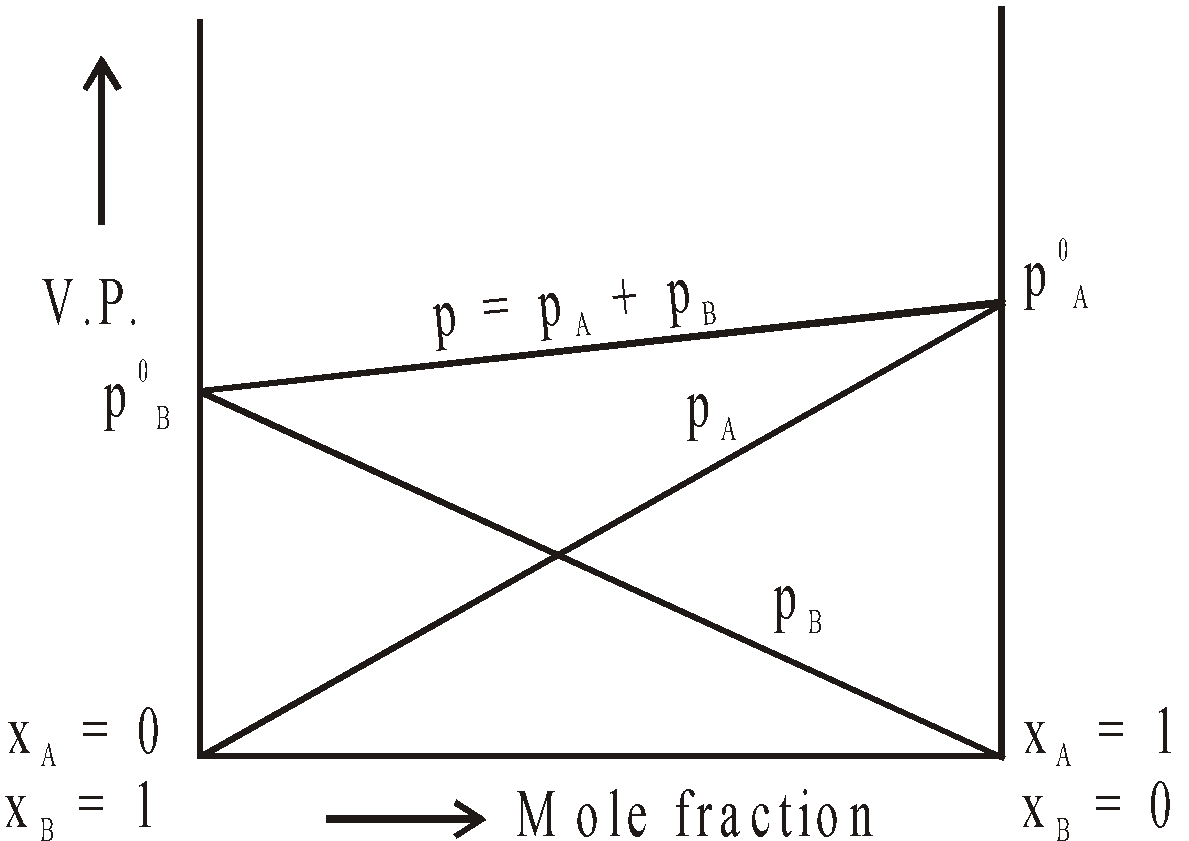Positive Deviation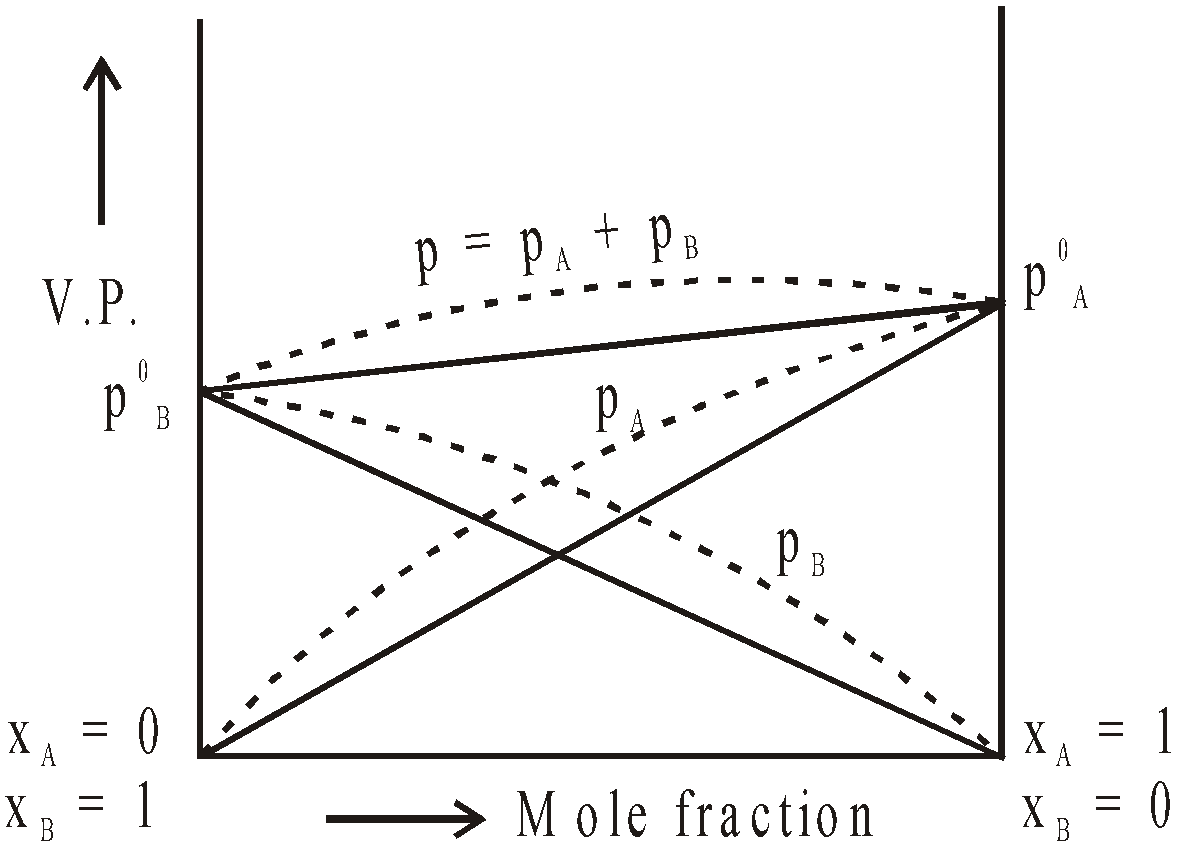Negative Deviation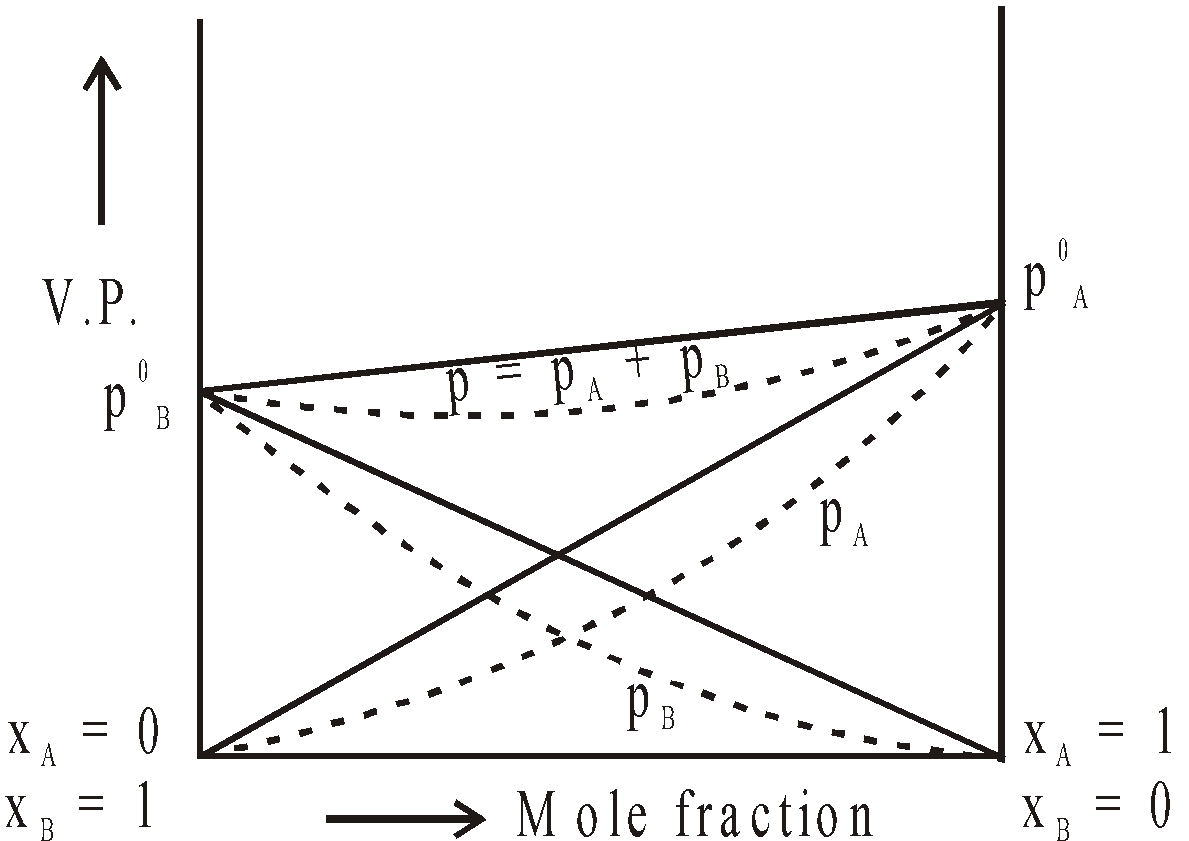### COLLIGATIVE PROPERTIES

Properties which depend upon the number of particles irrespective of their nature (ions or molecules) are called colligative properties. These are
• Relative lowering of vapour pressure
• Osmotic pressure
• Elevation in boiling point
• Depression in freezing point

### RELATIVE LOWERING OF VAPOUR PRESSURE

It is measured by Ostwald and Walker’s method (Gas saturation method)mole fraction of non volatile solute =w2 = loss in weight of solvent
w1 = loss in weight of solution

### OSMOSIS

The spontaneous flow of the solvent through a semipermeable membrane from pure solvent to solution or from a dilute solution to concentrated solution is known as osmosis.
Some natural semipermeable membranes are pig’s bladder, skin round white of an egg, membrane round the red blood corpuscle and in the cell of the plant.

Cupric ferrocyanide Cu2[Fe(CN)6] is artificially prepared semi-permeable membrane
(CuSO4 + K4 [Fe(CN)6])It is not suitable for non aqueous solution as it gets dissolved. Another artificial semipermeable membrane is Ca3(PO4)2.

### OSMOTIC PRESSURE (Π)

The hydrostatic pressure developed on the solution which just prevents the osmosis of pure solvent into the  solution through a semipermeable membrane is called osmotic pressure.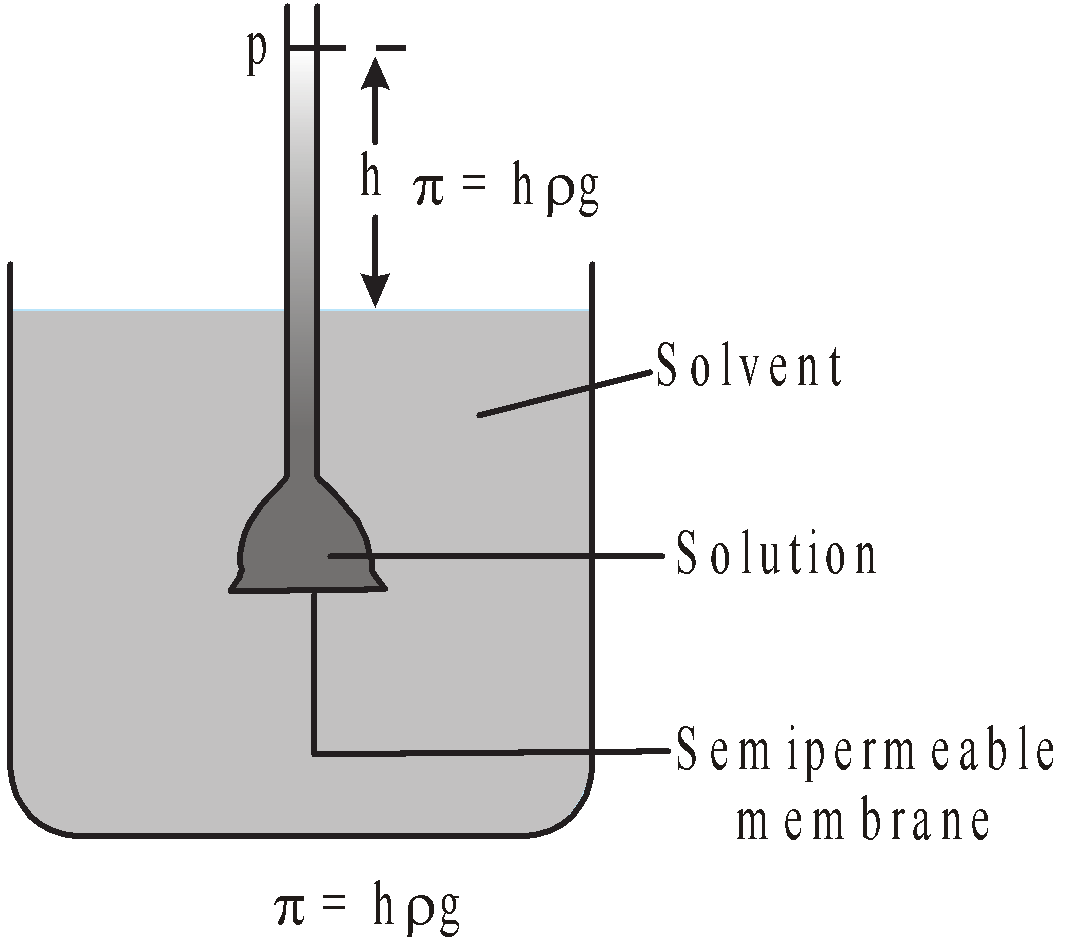=hg
or
The minimum external pressure applied to the solution to just stop the process of osmosis is known as osmotic pressure.or
The minimum external pressure applied on solution to make its vapour pressure equal to that of solvent is known as osmotic pressure.

#### DETERMINATION OF OSMOTIC PRESSURE

• Pfeffer’s method
• Berkeley and Hartley’s method (most common)
• Morse and Frazer’s method
• Townsend’s negative pressure method
• De. Vries method

Isotonic Solutions : Solutions having the same osmotic pressure.
0.91% NaCl solution is isotonic with human RBC’s
Hypotonic Solution : Solution having lower Osmotic pressure
Hypertonic Solution : Solution having higher osmotic pressure

### VAN’T HOFF’S THEORY OF DILUTE SOLUTIONS

Dilute solutions behave like gases and gas laws can be applied to them.

#### BOYLE-VAN’T HOFF LAW :

Osmotic pressure of a solution is directly proportional to its concentration at constant given temperature.#### CHARLE’S-VAN’T HOFF LAW

Osmotic pressure of a solution is directly proportional to its temperature at fixed concentration.#### VAN’T HOFF EQUATION FOR SOLUTIONSS = Solution constant having same value as gas constant
C = Molar concentration
T = Absolute temperature and p = Osmotic pressure

#### AVOGADRO-VAN’T HOFF LAW FOR SOLUTIONS

Equal volumes of solutions having the same osmotic pressure and temperature contain equal number of moles i.e. have same concentration.

#### RELATION BETWEEN OSMOTIC PRESSURE (P) AND LOWERING OF VAPOUR PRESSURERelative lowering of vapour pressure is directly proportional to osmotic pressure

### OSMOLARITY

Osmotic behaviour of solutes undergoing association or dissociation is equal to molarity × number of particles produced per formula unit of solute known as osmolarity.

### REVERSE OSMOSIS

When the external pressure applied on the solution is more than osmotic pressure, the solvent flows from the solution to the pure solvent which is called reverse osmosis. Desalination of seawater is done by reverse osmosis to make it potable.

### PLASMOLYSIS

The flow of the fluid from the plant cell when placed in a hypertonic solution is called plasmolysis. The plant cell undergoes shrinkage.

### EXO-OSMOSIS

Outward osmotic flow of fluid from a cell through semipermeable membrane e.g. grape in conc. NaCl solution.

### ENDO-OSMOSIS

Inward osmotic flow of fluid from a cell through semipermeable membranes e.g. grape in water.

### SILICA GARDEN

When coloured salts e.g. Copper Sulphate, Cobalt Nitrate, Manganese Chloride, Nickel Chloride and Ferrous sulphate etc. are placed in Sodium silicate solution of density 1.1, a colloidal and semipermeable shell of Silicates is formed round the crystal. Inside this, there is strong solution of salt and outside weak solution of sodium silicate. Water permeates into the shell, pressure rises and the shell bursts out, the salt solution escapes but immediately reacts with sodium silicate to form again semipermeable shell of the metallic silicate. The process is repeated again and again and projection of coloured silicates continues to grow.

### KONOWALOFF’S RULE

The mole fraction of the more volatile component is always greater in the vapour phase than in the solution phase.

### RELATION BETWEEN THE TOTAL PRESSURE AND MOLE FRACTIONS OF VOLATILE COMPONENTS IN LIQUID PHASE### RELATION BETWEEN THE TOTAL PRESSURE AND MOLE FRACTIONS OF VOLATILE COMPONENTS IN VAPOUR PHASE### BOILING POINT AND ELEVATION IN BOILING POINT

The temperature at which the vapour pressure of a liquid becomes equal to atmospheric pressure is known as the boiling point of the liquid.
Addition of non volatile solute in a solvent lowers the vapour pressure and increases the boiling point. The increase in boiling point is directly proportional to the molality of solution.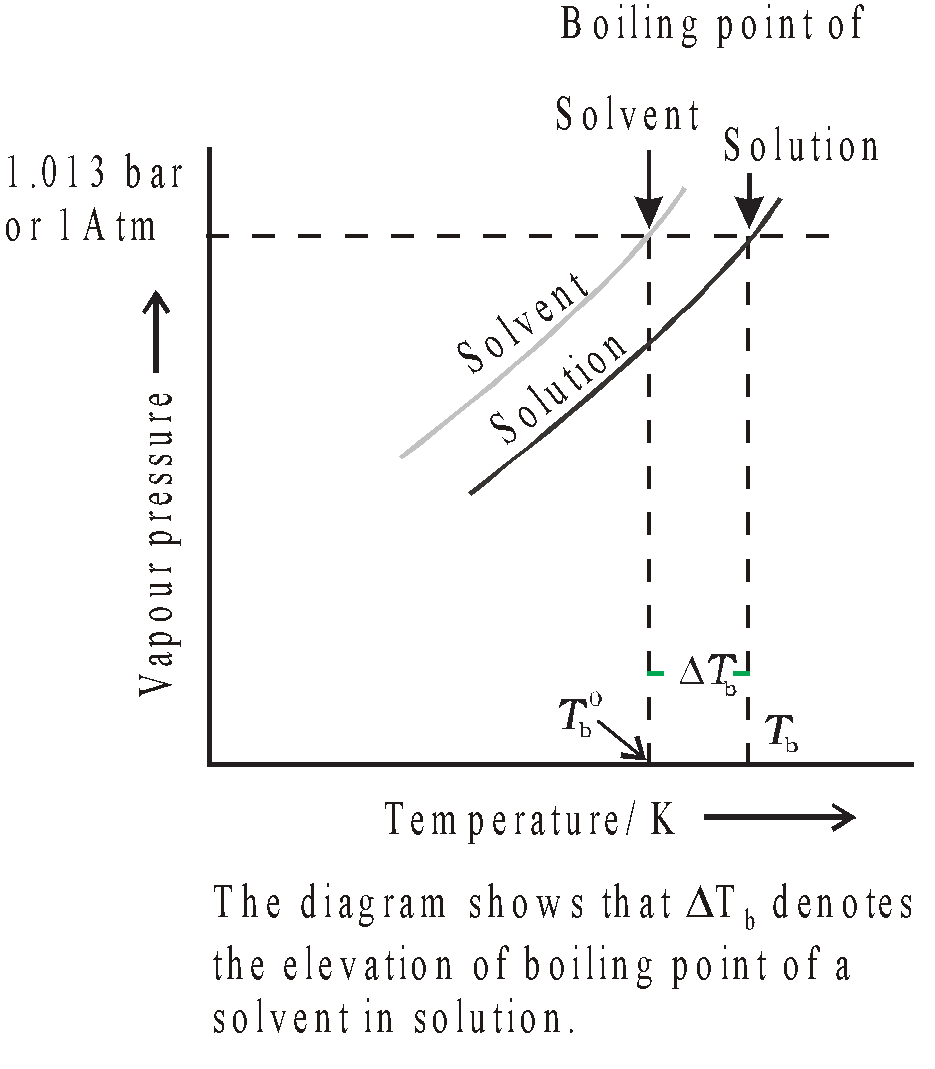Kb = molal elevation constant or ebullioscopic constant. It is characteristic for given solvent. When
m = 1,Tb = Kb.

Thus molal elevation constant or ebullioscopic constant is defined as the elevation in boiling point caused by one molal solution. Its value is given byUnits of  Kb = K kg mol–1
R = gas constant, T0 = normal boiling point  lv = latent heat of vaporisation per gram of pure solvent. Kb is constant for given solvent.
MA = molecular mass of solvent, Enthalpy of vaporisation of solvent

#### MOLAL ELEVATION CONSTANTS OF SOME COMMON SOLVENTS

 Solvents Value of Kb in K kg mol–1 Water 0.52 Methyl alcohol 0.80 Acetone 1.72 Benzene 2.53 Carbon tetrachloride 5.03 Chloroform 3.63 Ethyl alcohol 1.20 Ethyl ether 2.11

#### DETERMINATION OF MOLECULAR WEIGHT FROM BOILING POINT ELEVATIONKb = molal elevation constant,
w = weight of solute,
W = weight of solvent,
DTb = Elevation in bpt.

The method employed is known as ebullioscopic method. The common methods are
• Landsberger’s method
• Coltrell’s method

### FREEZING POINT AND DEPRESSION IN FREEZING POINT

The temperature at which the vapour pressure of the solvent in its liquid and solid phase becomes the same is known as freezing point of solvent.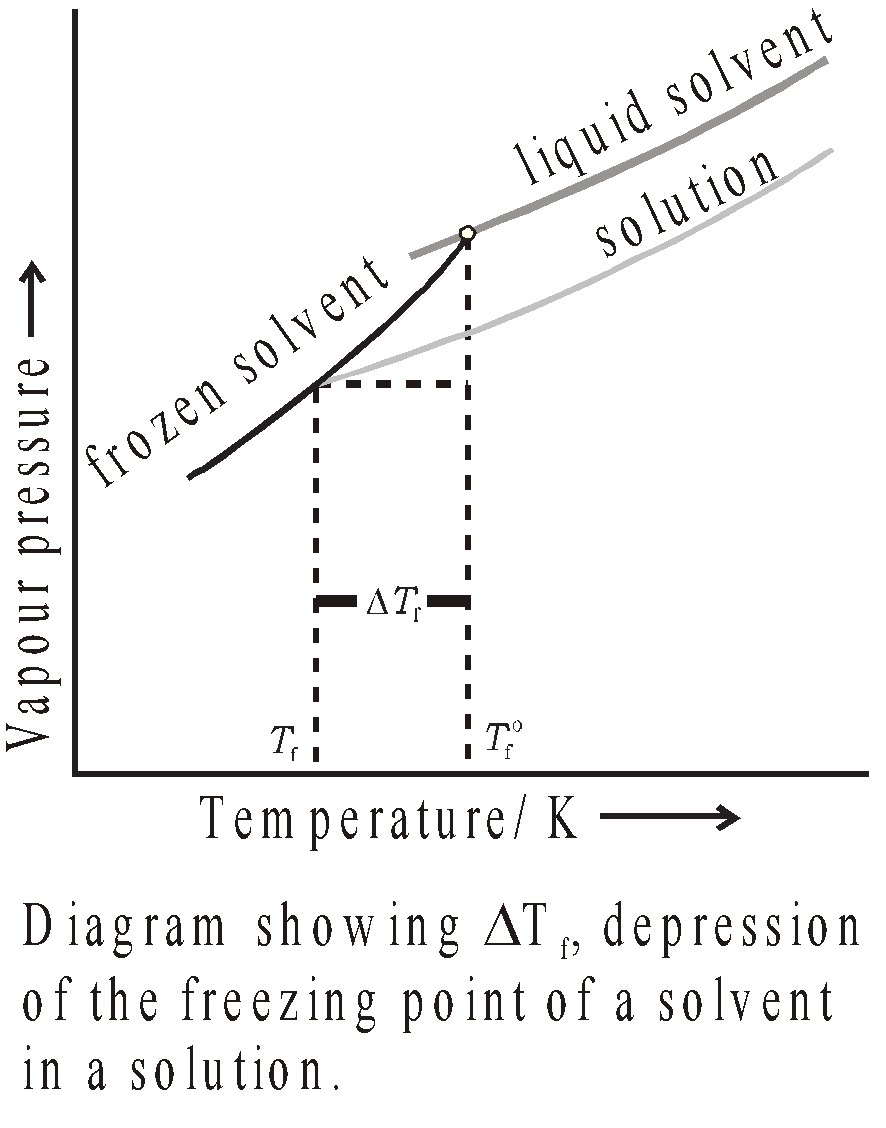When non volatile solute is dissolved in a solvent, the freezing point decreases. Depression in freezing point is given byKf is molal depression constant or molal Cryoscopic constant.
When m (molality) = 1 thenHence molal depression constant or molal Cryoscopic constant is equal to depression in freezing point caused by one molal solution. Its value is given byUnits of Kf = K . Kg mol–1
R = gas constant,
T0 = normal freezing point,
lf = heat of fusion per gram of solvent.
Kf is freezing point constant for a given solvent.

#### MOLAL DEPRESSION CONSTANTS OF SOME COMMON SOLVENTS

 Water 1.86 Naphthalene 6.9 Acetic acid 3.9 Bromoform 14.4 Phenol 7.27 Cyclohexane 20 Benzene 5.12 Camphor 37.7

#### DETERMINATION OF MOLECULAR WEIGHT BY DEPRESSION IN FREEZING POINTKf = Molal depression constant,
w = weight of non-volatile solute,
ΔTf = depression in freezing point,
W = weight of solvent

The method employed is known as Cryoscopic method. The common methods are
• Beckmann method
• Rast Method

### RELATION BETWEEN OSMOTIC PRESSURE AND OTHER COLLIGATIVE PROPERTIESRelative lowering of vapour pressureElevation in boiling pointDepression in freezing point
π = Osmotic pressure,
d = Density of solution at temperature T,
R = Gas constant,
MB = Molecular mass of solute,
Kb = Molal elevation constant of solvent,
Kf = Molal depression constant of solvent.

### ABNORMAL MOLECULAR MASS AND COLLIGATIVE PROPERTIES

When solutes undergo association or dissociation in solution, there is decrease or increase in number of particles and there are discrepancies between observed and calculated values of colligative properties.
Colligative property, hence higher values are obtained for molecular masses in case of association.
Acetic acid in benzene associates and its observed molecular mass is 120.
Benzoic acid in benzene associates and its observed molecular mass is 244.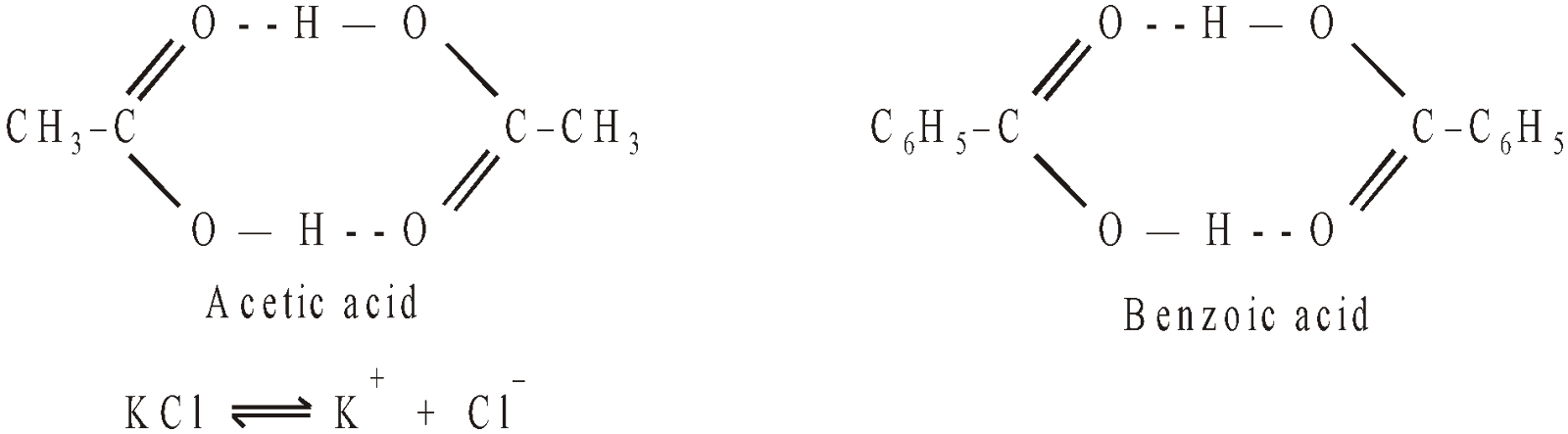KCl in water dissociates and its observed molecular mass is 37.25.

### VAN’T HOFF FACTOR (i )

Its value is obtained by any of the following expression
• i =• i =• i =#### DEGREE OF DISSOCIATION

Fraction of total number of molecules undergoing dissociation,m = number of particles of electrolyte given in solution.
i = Van’t Hoff factor i.

#### DEGREE OF ASSOCIATION

Fraction of the total number of molecules undergoing association.### RELATION BETWEEN RELATIVE LOWERING OF VAPOUR PRESSURE AND MOLALITY OF SOLUTIONWhere m = molality of solution
M1 = Molecular weight of solvent

### RELATION BETWEEN WEIGHTS OF TWO IMMISCIBLE LIQUIDS IN THE DISTILLATEwhere w = weight of liquid
p = partial pressure
M = Molecular weight

### CONJUGATE SOLUTIONS

When two partially miscible liquids A and B are mixed with each other, two layers are formed. First, Solution of A in B and Second, Solution of B in A. Such solutions coexisting together are called conjugate solutions. For example solution of phenol and water.

### CRITICAL SOLUTION TEMPERATURE OR CONSOLUTE TEMPERATURE

On heating the conjugate solution the mutual solubility of A and B increases. A temperature is reached when two phases disappear and one homogeneous phase is left. This temperature is known as Critical solution temperature or Consolute Temperature. It is of two types :

Upper Consolute temperature : For example water-phenol system.

Lower Consolute temperature : In some cases it is also possible to have a lower temperature of similar kind. For example- Trimethylamine-water is a lower consolute temperature.